Next: The Lorentz Force Up: Magnetism Previous: Ampère's Experiments

## Ampère's Law

Magnetic fields, like electric fields, are completely superposable. So, if a field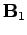is generated by a current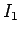flowing through some circuit, and a field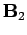is generated by a current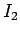flowing through another circuit, then when the currentsandflow through both circuits simultaneously the generated magnetic field is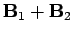. This is true at all points in space.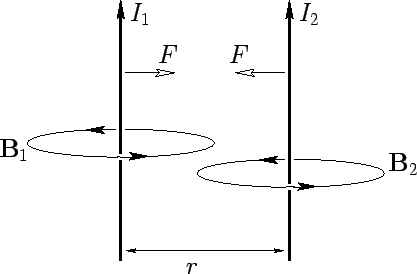Consider two parallel wires separated by a perpendicular distance, and carrying electric currentsand, respectively. The magnetic field-strength at the second wire due to the current flowing in the first wire is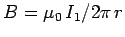. This field is orientated at right-angles to the second wire, so the force per unit length exerted on the second wire is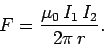(156)

This follows from Eq. (152), which is valid for continuous wires as well as short test wires. The force acting on the second wire is directed radially inwards towards the first wire. The magnetic field-strength at the first wire due to the current flowing in the second wire is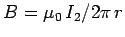. This field is orientated at right-angles to the first wire, so the force per unit length acting on the first wire is equal and opposite to that acting on the second wire, according to Eq. (152). Equation (156) is called Ampère's law.

Incidentally, Eq. (156) is the basis of the official SI definition of the ampere, which is:

One ampere is the magnitude of the current which, when flowing in each of two long parallel wires one meter apart, results in a force between the wires of exactly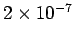N per meter of length.
We can see that it is no accident that the constant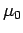has the numerical value of exactly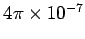. The SI system of units is based on four standard units: the meter, the kilogram, the second, and the ampere. Hence, the SI system is sometime referred to as the MKSA system. All other units can be derived from these four standard units. For instance, a coulomb is equivalent to an ampere-second. You may be wondering why the ampere is the standard electrical unit, rather than the coulomb, since the latter unit is clearly more fundamental than the former. The answer is simple. It is very difficult to measure charge accurately, whereas it is easy to accurately measure electric current. Clearly, it makes sense to define a standard unit in terms of something which is easily measurable, rather than something which is difficult to measure.Next: The Lorentz Force Up: Magnetism Previous: Ampère's Experiments
Richard Fitzpatrick 2007-07-14